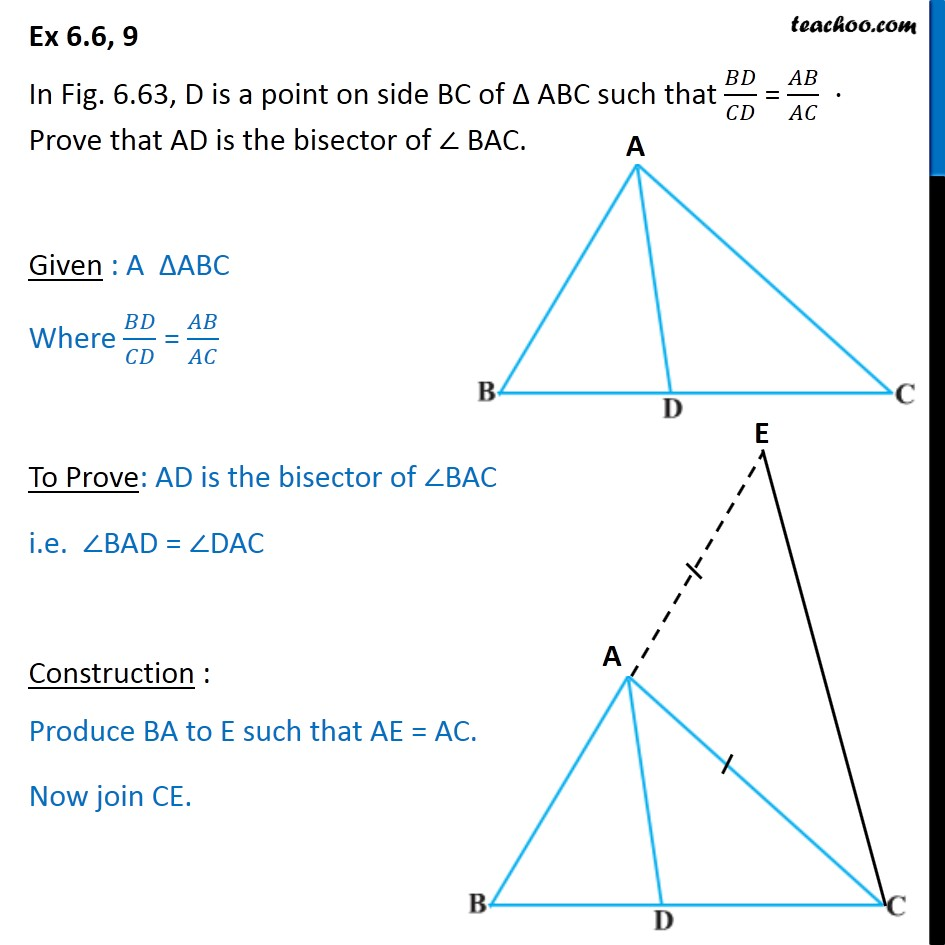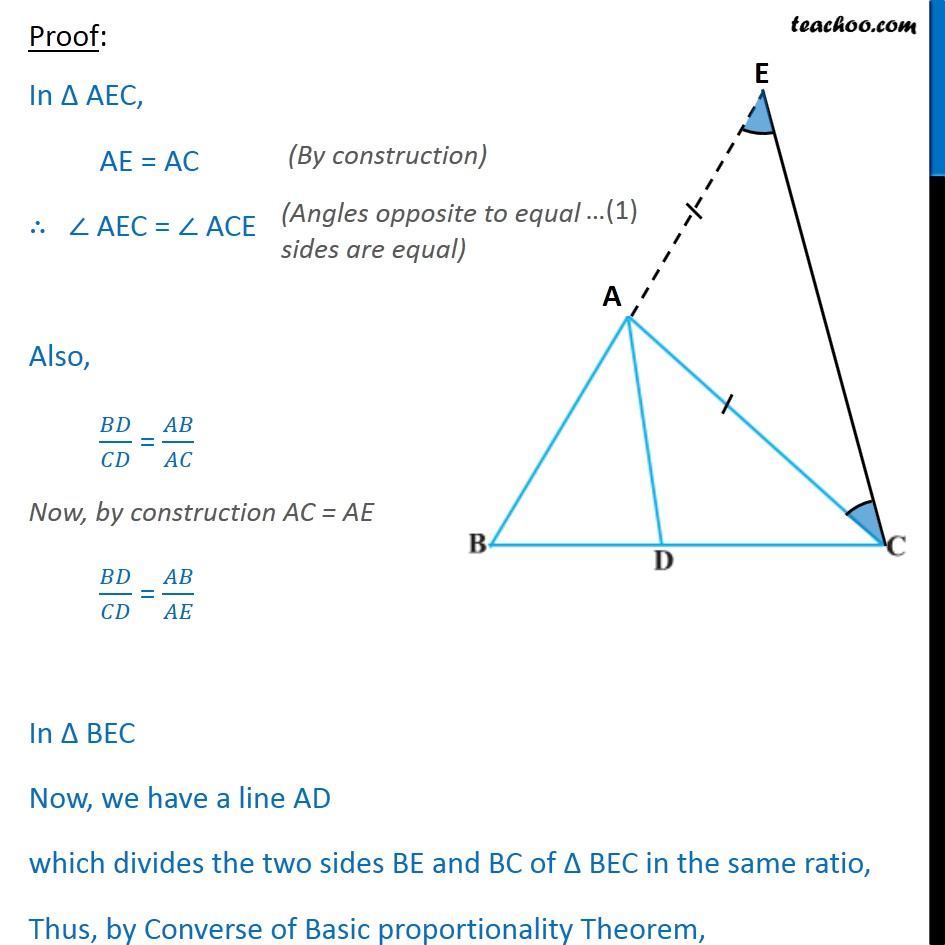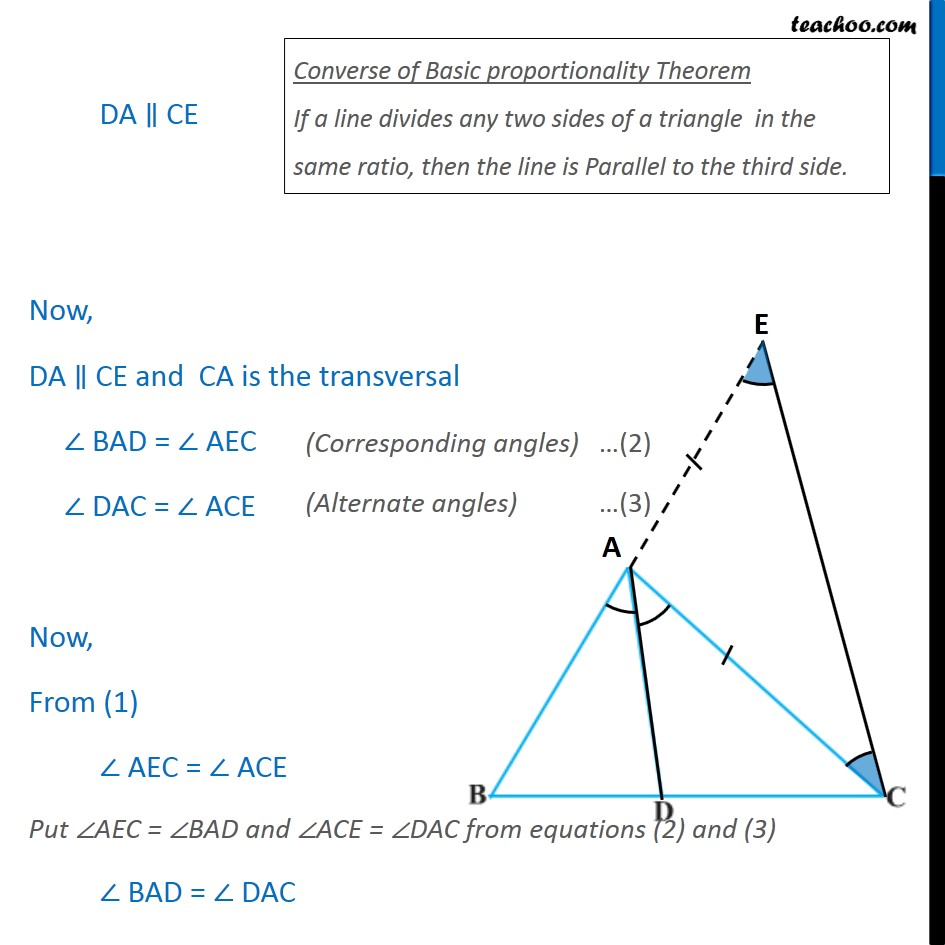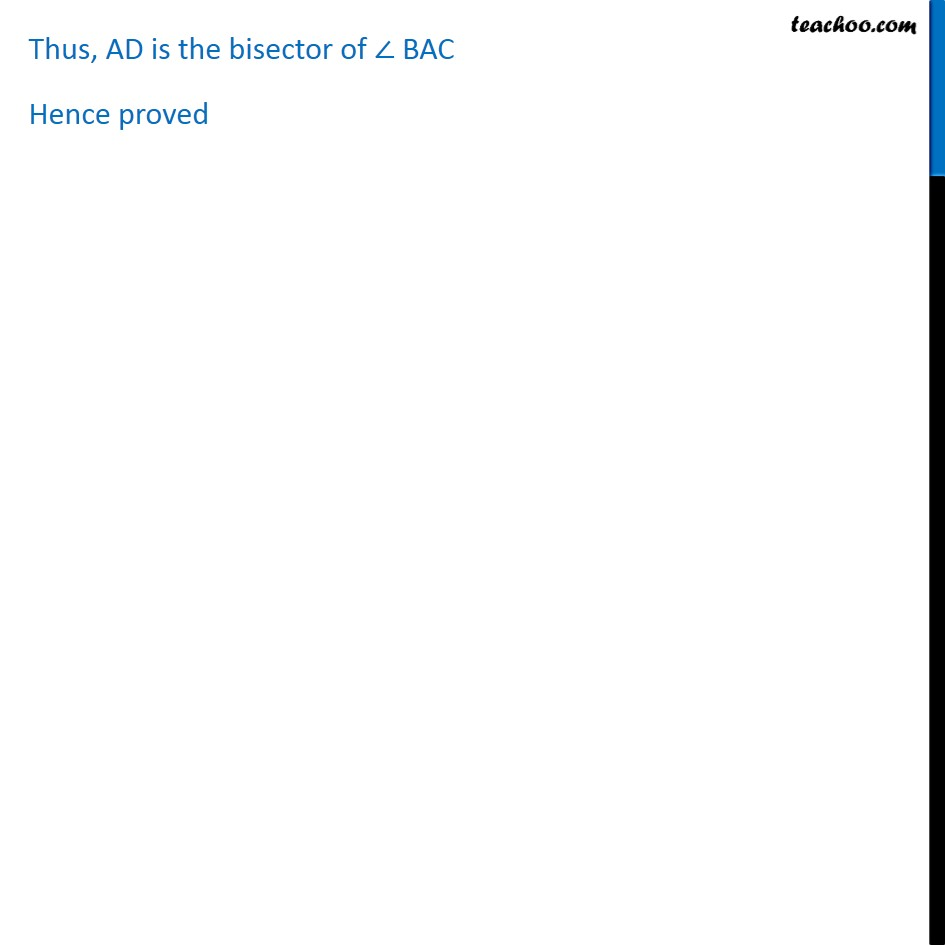Important questions of Triangle (in Geometry)

Chapter 6 Class 10 Triangles
Serial order wiseLearn in your speed, with individual attention - Teachoo Maths 1-on-1 Class

### Transcript

Question 9 In Fig. 6.63, D is a point on side BC of ABC such that / = / Prove that AD is the bisector of BAC. Given : A ABC Where / = / To Prove: AD is the bisector of BAC i.e. BAD = DAC Construction : Produce BA to E such that AE = AC. Now join CE. Proof: In AEC, AE = AC AEC = ACE Also, / = / Now, by construction AC = AE / = / In BEC Now, we have a line AD which divides the two sides BE and BC of BEC in the same ratio, Thus, by Converse of Basic proportionality Theorem,DA CE Now, DA CE and CA is the transversal BAD = AEC DAC = ACE Now, From (1) AEC = ACE Put AEC = BAD and ACE = DAC from equations (2) and (3) BAD = DAC Converse of Basic proportionality Theorem If a line divides any two sides of a triangle in the same ratio, then the line is Parallel to the third side. Thus, AD is the bisector of BAC Hence proved